# J. Antoni’s Fast Spectral Correlation Estimator

The Fast Spectral Correlation estimator is a quick way to find small cycle frequencies. However, its restrictions render it inferior to estimators like the SSCA and FAM.

In this post we take a look at an alternative CSP estimator created by J. Antoni et al (The Literature [R152]). The paper describing the estimator can be found here, and you can get some corresponding MATLAB code, posted by the authors, here if you have a Mathworks account.

I should say that I don’t think there is much wrong with the paper–the presented spectral correlation estimator is fast in some settings. But of course that isn’t the whole story.

### The Fast Spectral Correlation (FSC) Estimator

The stated motivation for developing the FSC is the high computational cost, and therefore processing delay, of both the FFT accumulation method (FAM) and the time-smoothing method (TSM) of spectral correlation and spectral coherence estimation. The problem isn’t the TSM itself, but the act of putting the TSM in a loop over cycle frequency to find the significant cycle frequencies in some data record. Putting the frequency-smoothing method (FSM) in a loop is also conceptually attractive as a way to find cycle frequencies. As we already know, the SSCA and FAM are much more efficient (though still costly) for cycle-frequency searches than either the FSM-in-a-loop or the TSM-in-a-loop.

After you read this post, if you are still interested in the FSC, I encourage you to study the source material in [R152]. Here I am going to provide the definition of the FSC mathematically, but I’m not going to rehash all the mathematical development in [R152] that leads to the definition.

Let’s suppose our data is represented by the time-series$x(t)$ which is a discrete-time sequence (typically real-valued for Antoni) with length$L$. The FSC will involve the discrete Fourier transform of a product of short-time Fourier transforms (like those we see in our definition of the TSM). The block length for the short-time Fourier transforms is denoted by$N_w$. The temporal spacing between adjacent$N_w$-sample blocks is denoted by$R$ samples. For example, non-overlapping adjacent blocks use$R = N_w$. Then the FSC is defined by$\displaystyle S_x^{fast}(\alpha, f) = \frac\sum_{p=0}^P S_x(\alpha, f; p)}\sum_{p=0}^P R_w(\alpha - p\Delta f)} R_w(0) \hfill (1$

where$\displaystyle S_x(\alpha, f; p) = \frac{1}{K\| w \|^2 f_s} {\mbox{\rm DFT}}_{i\rightarrow\alpha} \left\{X_T(i, f) X_T(i, g)^* \right\} e^{-i 2 \pi N_0 \left( \frac{\alpha}{f_s} - \frac{p}{N_w} \right)}, \hfill (2)$

and$\displaystyle X_T (i, f) = \sum_{m=0}^{N_w-1} x(iR+m) w(m) e^{-i 2 \pi m f / f_s}, \hfill (3)$$w(m)$ is a data-tapering window with length$N_w$,$\|w\|^2$ is the energy in the tapering window,$R_w(\cdot)$ is$\displaystyle R_w(\beta) = \sum_{n=0}^{N_w-1} |w(n)|^2 e^{-i 2 \pi (n-N_0)\beta/f_s}, \hfill (4)$$f = k\Delta f$,$g = (k-p)\Delta f$,$N_0$ is the midpoint of the window$w(\cdot)$ (unfortunately named, since$N_0$ is universally used for the spectral density of white Gaussian noise),$\Delta f = f_s/N_w$, and the integer$P = \lfloor N_w/2R \rfloor$.

Antoni shows that this estimate converges ($L \rightarrow \infty, N_w \rightarrow \infty$) to the spectral correlation function for cycle frequencies that are below the maximum given by$\displaystyle \alpha_{max} = f_s/(2R). \hfill (5)$

Since$R \ge 1$,$\alpha_{max} \leq f_s/2$.

So one can see that the FSC is a Fourier transform of a sequence of cyclic periodograms, or things very similar to cyclic periodograms: products of frequency-shifted Fourier transforms, possibly phase-compensated.

### The Drawback of the FSC

The problem with the FSC is that it is only fast when the maximum considered cycle frequency is a small fraction of the sampling rate. Moreover, the maximum value of cycle frequency for which one can obtain a spectral correlation estimate must be smaller than half the sampling rate.

#### Small Maximum Cycle Frequency

Antoni’s example considers a synthetic (meaning “not captured data” or “simulated”) cyclostationary signal sampled at$f_s =$ 1 kHz. In the provided implementation and example code, the maximum cycle frequency is set to 40 Hz. The number of processed samples is very close to$2^{17} = 131072$, so that the cycle-frequency resolution of any estimator is approximately$\displaystyle \Delta\alpha \approx 1/L = 1000/131072 = 0.008 \mbox{\rm \ Hz}$.

For TSM or FSM in a loop over cycle frequency, we’d want to visit cycle frequencies on the interval$[0, 40]$ in steps of about$0.008$ Hz, or about$40/0.008 = 5000$ cycle frequencies. But the principal domain of the spectral correlation function is a diamond-shaped region in the$f-\alpha$ plane with vertices$(f, \alpha) = \{(-fs/2, 0), (0, f_s), (f_s/2, 0), (0, -f_s)$. For the non-conjugate spectral correlation function, some of this principal domain is redundant, but none is for the conjugate spectral correlation function. The traditional one-dimensional principal domain for digital signal processing in the frequency domain ($[-f_s/2, fs_2])$) is subsumed into this two-dimensional cyclic principal domain. So the stated restriction to a “small” cycle frequency means a great many cycle frequencies potentially exhibited by the data under study will go undetected.

The restriction on the maximum cycle frequency that says it should be small relative to$f_s$ isn’t because the algorithm won’t work for larger cycle frequencies, it is because the performance (computational cost) of the FSC is superior to some other methods when the maximum considered cycle frequency is small. When you specify a larger maximum cycle frequency, the algorithm (as Antoni has coded it) adjusts$R$ accordingly. But once$R$ becomes too small (the maximum cycle frequency too large), the FSC becomes quite slow compared to alternatives.

And, as we will see, a good implementation of the SSCA or FAM can produce estimates of all significant cycle frequencies in less time than the FSC can produce estimates of significant cycle frequencies for its highly restricted cycle-frequency range!

#### Limiting the Cycle Frequency to$f_s/2$$f_s/2$

The$(f, \alpha)$ principal domain is important because it tells us the maximum possible non-conjugate cycle frequency is$\alpha = f_s$. This isn’t an academic point. In the application of CSP to communication signals, an important cycle frequency can easily exceed$f_s/2$ and be near$f_s$. An example (which we look at below) is a PSK or QAM signal that is only slightly oversampled (the sample rate is slightly greater than the occupied bandwidth). Unlike the restriction based on desiring very fast computational, this restriction is a must–Antoni’s code will not run if the maximum cycle frequency is set equal to$f_s/2$ or higher.

This restriction complicates the application of the FSC to communication signals and RF scenes. And, to be fair, that is not Antoni’s interest–he is looking at the link between a fault in a mechanical system that involves rotating parts (for example, bearings) and the cyclostationarity of signals associated with the mechanical system. Imminent faults may be diagnosable earlier by using CSP compared to traditional fault-detection methods in that field.

A common situation for us in communication-signal processing (and that is the stated area of interest of the CSP Blog: Understanding and Using the Statistics of Communication Signals) is processing a complex time-series that arises from the detection of a signal, or signals, in some frequency band of interest using energy detection or spectrum estimation methods. For example, Figure 1 shows the PSD for a simulated BPSK signal in noise that has been extracted from a more wideband scene.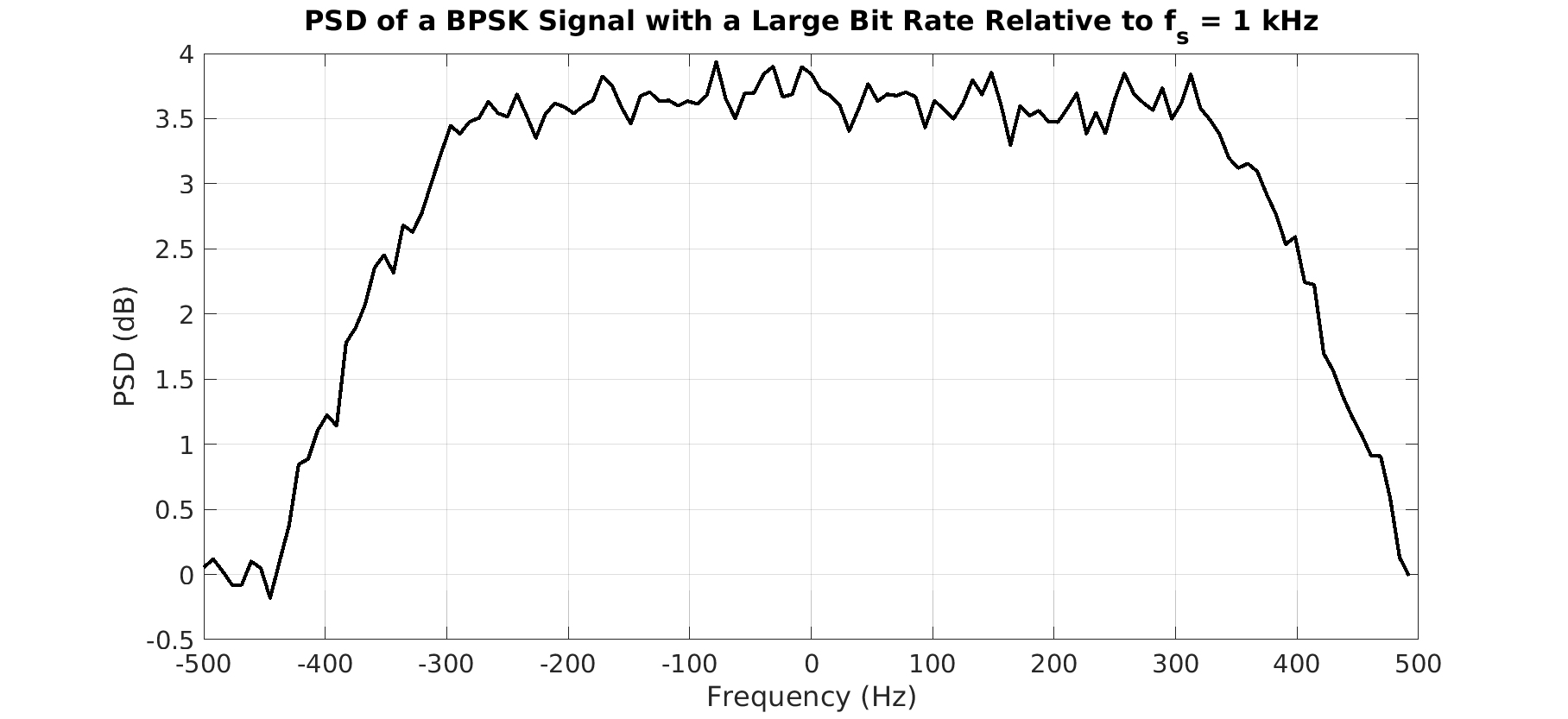Figure 1. Estimated PSD for a BPSK signal with a bit rate of Hz and sampled at kHz. Therefore, its single non-conjugate cycle frequency is Hz, which is much greater than Hz.

Antoni’s code won’t be useful for such data as in Figure 1, because the unknown cycle frequency is much greater than half the sampling rate. However, we can easily apply the FAM, SSCA, FSM, or TSM to this data to find all its cycle frequencies. The elapsed time, mirroring the number of required multiplications, will vary considerably over these four basic spectral-correlation estimators. The results for applying the SSCA are shown in Figure 2. To process$131072$ samples of this high-rate BPSK signal using the straightforward (no parallelization, algorithm hacks, etc.) SSCA (non-conjugate) takes about 0.6 seconds.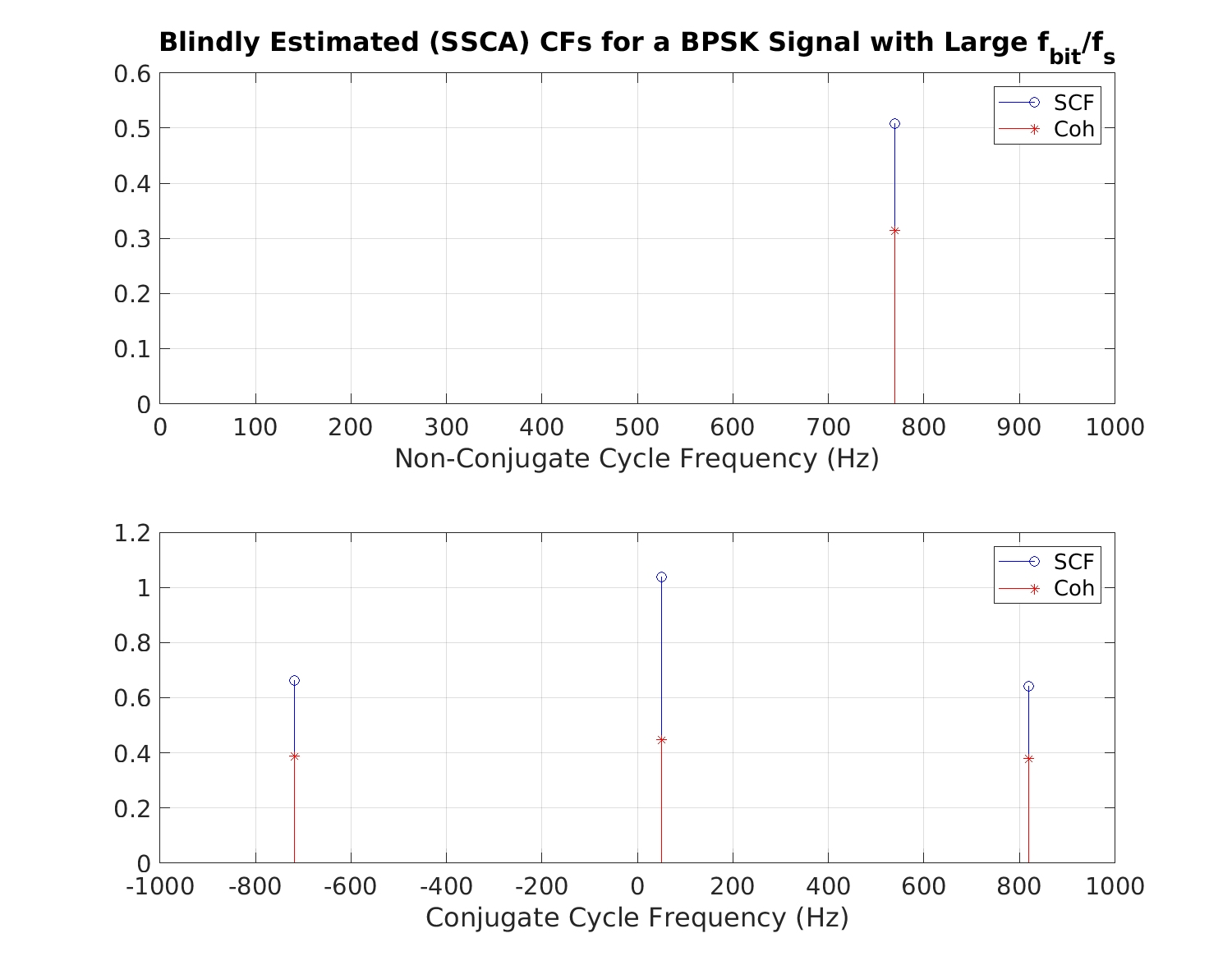Figure 2. SSCA-produced cycle frequencies for the high-rate BPSK signal with PSD shown in Figure 1. This signal cannot be successfully processed by Antoni’s FSC due to the large cycle frequencies relative to the sampling rate.

But let’s get to the point. In the following, I provide timing results for the SSCA, FAM, FSM-in-a-loop, TSM-in-a-loop, and the FSC for Antoni’s test input. I also show some output results to give the reader some confidence that the elapsed times I’m presenting correspond to correct estimator outputs.

### Timing Tests

To reset, we are going to use the signal generated by Antoni’s sample FSC code, which has cycle frequencies of$10$ and$20$ Hz. It is real-valued data. It is not a communication signal. Here is the code for generating the signal:

% Synthesis of an elementary cyclostationary signal
% =================================================
Fs = 1e3;               % sampling frequency (in Hz)
L = 131072;             % signal length (number of samples)
f0 = .01*Fs;            % cycle frequency (in Hz)

x = randn(L,1);

% filtration by a resonance
a = [1 -2*cos(2*pi*.2)*.9 .9^2];
x = filter(1,a,x);

% periodic amplitude modulation
x = x.*(1 + sin(2*pi*(0:L-1)'*f0/Fs));

% addition of white noise (SNR = 0dB)
x = x + std(x)*randn(L,1);


We are going to process$131072$ samples and the sampling rate is$1$ kHz. The spectral resolution of all of the estimators is approximately$\Delta f \approx f_s/64 = 15.6$ Hz and of course the cycle-frequency resolution is fixed at$\Delta \alpha \approx 1/L = f_s/131072 \approx 0.008$ Hz. The elapsed time includes the time required to compute the spectral correlation and the spectral coherence. For the SSCA and FAM, it also includes the time required to apply a coherence-based threshold and perform cycle-frequency binning to yield a minimal set of detected cycle frequencies.Table 1. Elapsed times for processing Antoni’s synthetic cyclostationary signal with various spectral correlation estimators. All estimators produce both the spectral correlation and spectral coherence. (*) Over seven seconds of the FAM’s elapsed time is due to an inefficient coherence calculation. The SSCA and FAM produce estimates of the spectral correlation and spectral coherence for all possible cycle frequencies here.

The FSC is indeed faster than the FSM-in-a-loop and the TSM-in-a-loop provided that the maximum visited cycle frequency is a very small fraction of the sampling rate (here$40/1000 = 0.04 = 4$%). However, we can estimate all significant cycle frequencies in less time than the restricted-CF FSC by simply using the SSCA. Moreover, once the maximum cycle frequency is increased to a still-small fraction of the sampling rate equal to$20$%, the FSC is no longer faster than the FSM-in-a-loop.

As promised, here are some results that confirm that the estimators are all working. First, let’s look at the power spectrum estimates produced by several of the estimators in Figure 3.Figure 3. Power spectral density estimates produced by the SSCA, FAM, FSM, and TSM for Antoni’s synthetic cyclostationary signal. Note the high degree of correspondence. Only positive frequencies are included because the signal is real-valued.

Now let’s look at the cyclic-domain profiles (CDPs) produced by the FSC, TSM, and FSM for the case where the maximum visited cycle frequency is set to$40$ Hz. The full CDPs are shown in Figure 4 and a zoomed-in version is shown in Figure 5.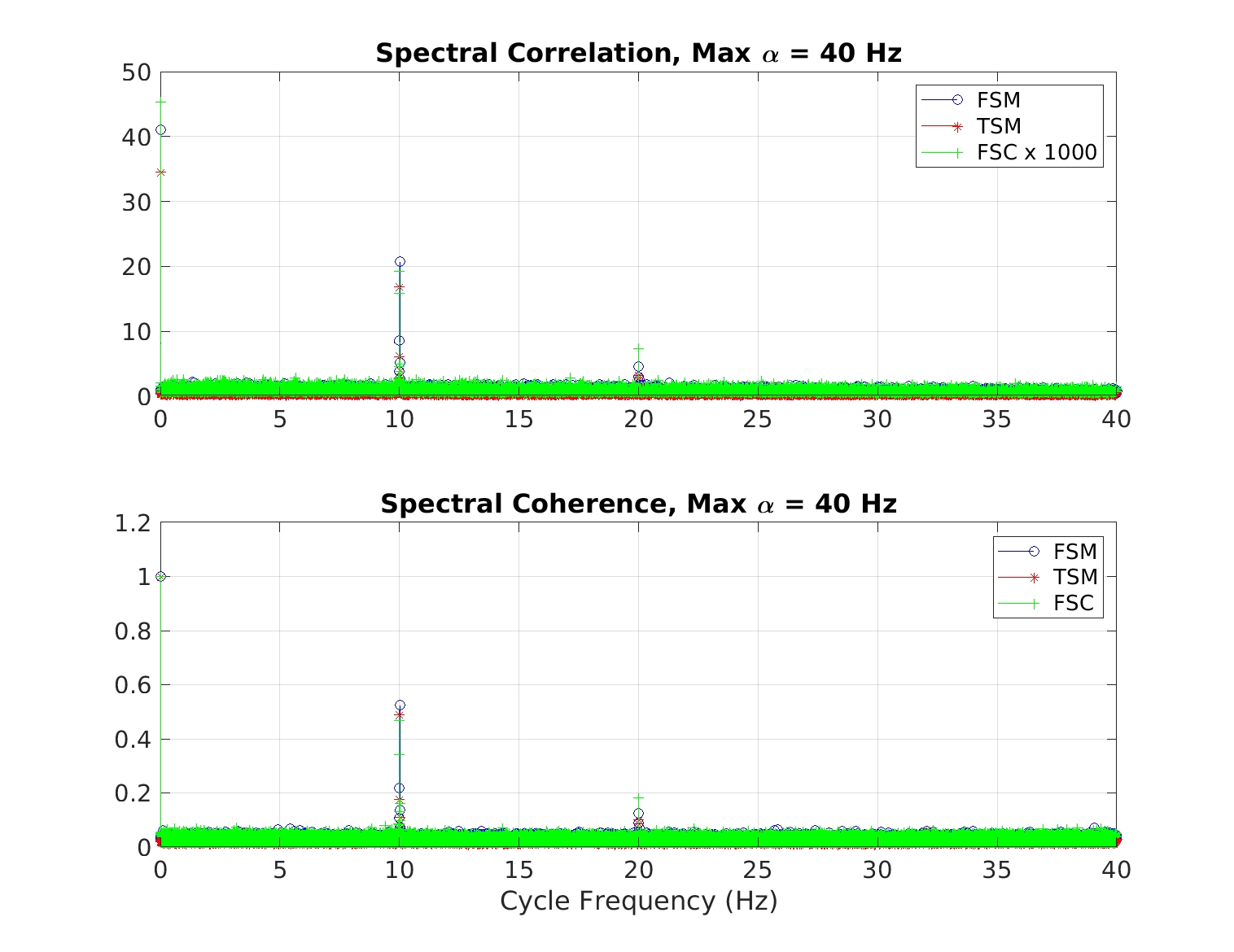Figure 4. Cyclic-domain profiles for three spectral correlation estimators applied to Antoni’s synthetic cyclostationary signal (true cycle frequencies at 10 and 20 Hz). The FSC produces very small spectral correlation estimates due to some scaling issue, so the spectral correlation values are multiplied by 1000 prior to plotting. Note the basic correspondence between the algorithms for both the two true cycle frequencies and all the false ones: The FSC works.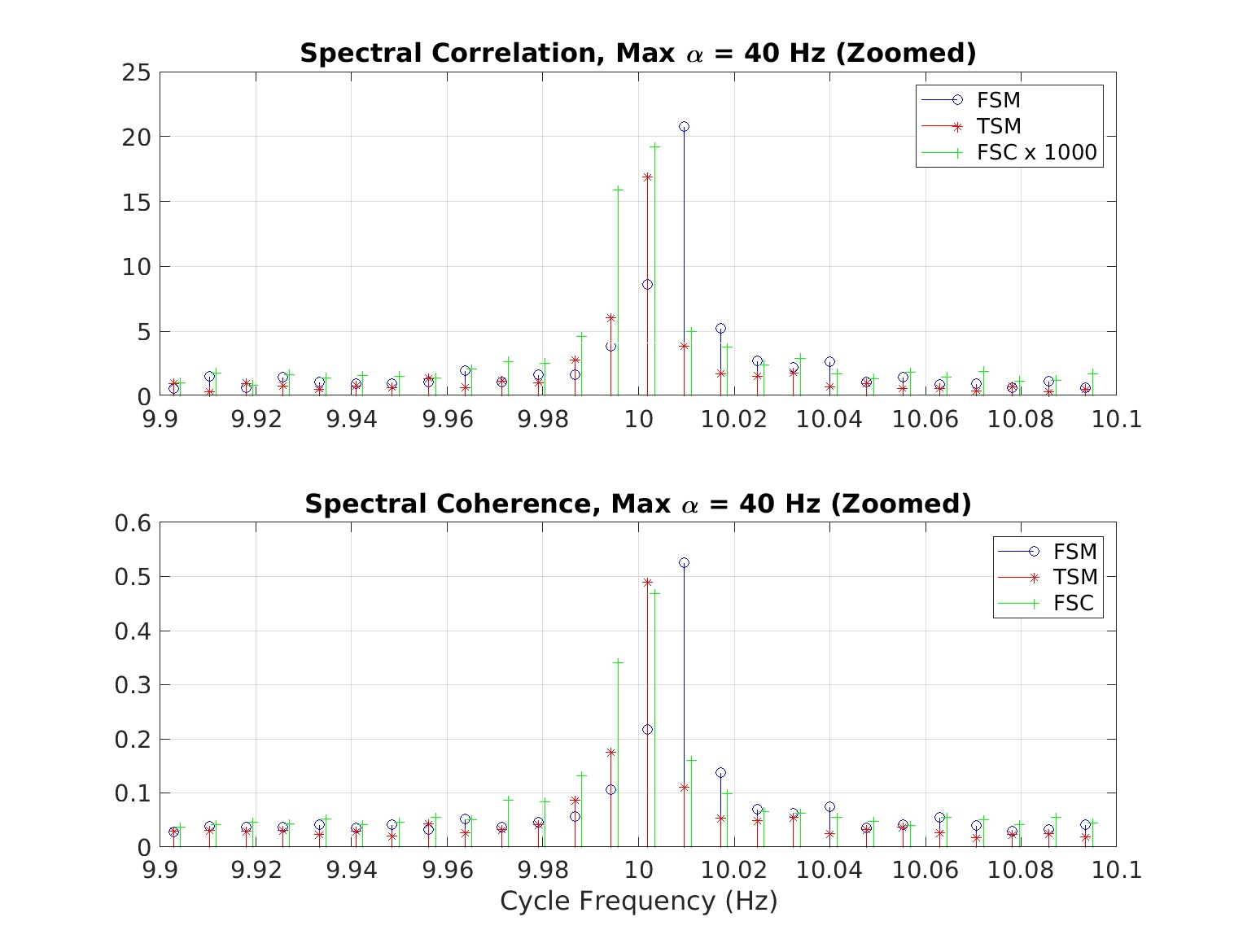Figure 5. Cyclic-domain profiles for three spectral correlation estimators applied to Antoni’s synthetic cyclostationary signal (true cycle frequencies at 10 and 20 Hz). This is a zoomed-in version of Figure 4. Note that all three algorithms produce a peak within about Hz of the true value of 10 Hz.

Finally, the CDPs for the three algorithms and a maximum visited cycle frequency of$200$ Hz are shown in Figure 6.Figure 6. Cyclic domain profiles for the FSC, FSM, and TSM for a maximum visited cycle frequency of Hz. Note the basic correctness and correspondence between the three algorithms.

Figures 4-6 give us good confidence that the elapsed-time numbers in Table 1 are fair to the three algorithms.

Turning to the FAM and SSCA, Figure 7 shows the CDPs produced by the SSCA and FAM for Antoni’s signal.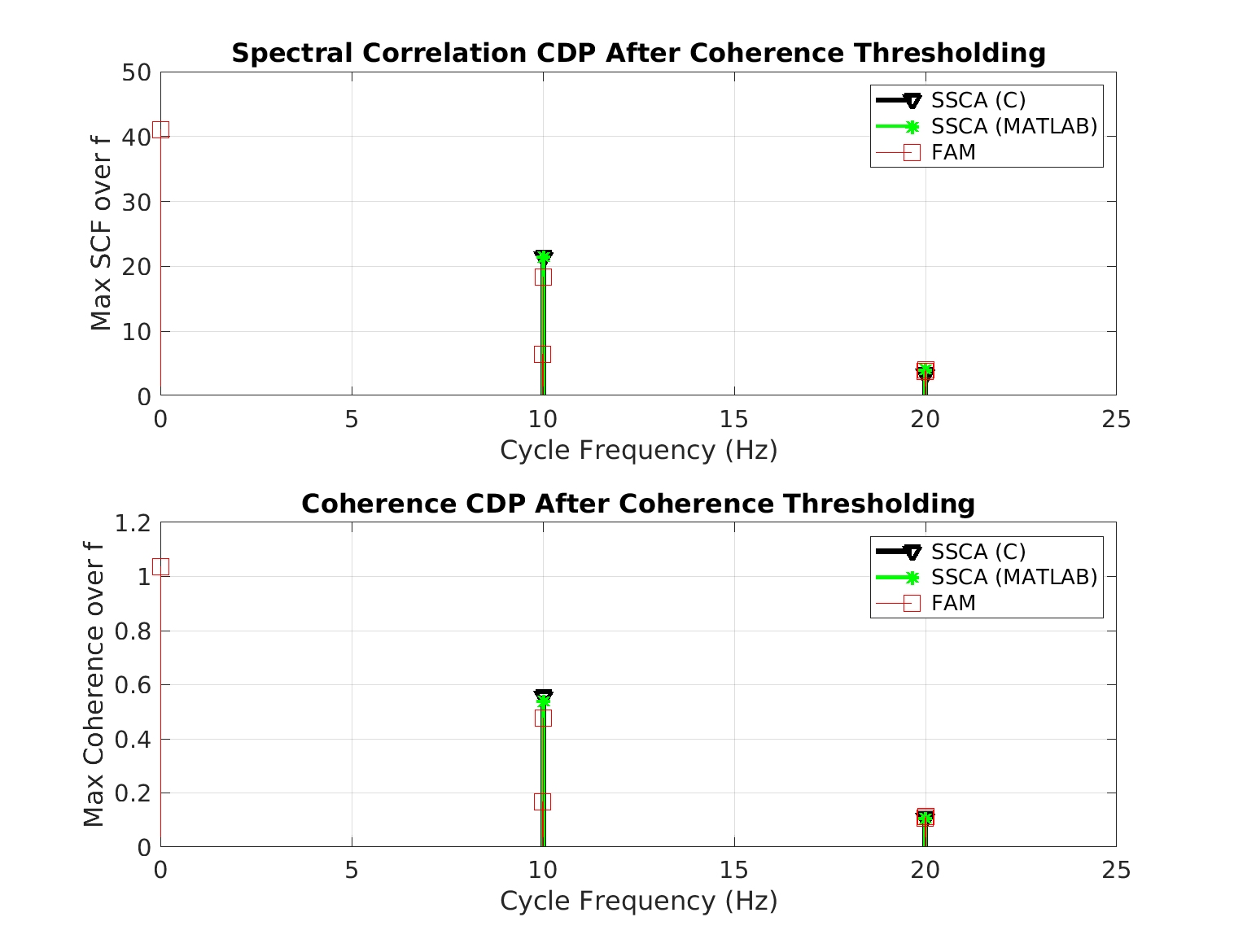Figure 7. Cyclic domain profiles produced by two versions of the SSCA and by the FAM for Antoni’s synthetic cyclostationary signal. Note the good correspondence in outputs. Also note that the FAM produces several detected cycle frequencies near the true value of Hz whereas the SSCA does not. The FAM typically suffers from relatively poor cycle leakage effects compared to the SSCA. However, these results indicate that the elapsed-time numbers in Table 1 are fair to the FSC.

Finally, all these algorithms can achieve smaller elapsed times by employing some form of parallelism. And some can achieve smaller elapsed times by employing algorithmic modifications that do not significantly reduce the probability of cycle-frequency detection for many input signal types of interest. As a small hint of this kind of development, I employed some parallelism for the SSCA and also employed strip decimation or selection. This just means that only some of the strips are computed (see the SSCA post for more details about strips). The results are shown in Figure 8.

The parallelism is denoted by the integer$P$ in Figure 8. Since the input data is real, the spectral correlation function for the first quadrant of the$(f, \alpha)$ plane is redundant with the other three quadrants–those three quadrants need not be considered in the selection of the strips to compute. Additionally, we can choose to visit only every$M$th strip (“strip decimation”). If the signal in the data is sufficiently wideband relative to the sampling rate, we have a low probability of missing its features if we decimate.

Figure 8 shows we can reduce the SSCA run time to 0.13 seconds using these tricks, which are highly applicable to Antoni’s signal.

### Conclusions

Antoni et al have developed the fast spectral correlation (FSC) estimator in The Literature [R152]. This estimator resembles the Fourier transform of a sequence of cyclic periodograms. A major drawback of the FSC is that there is always a restriction on the largest cycle frequency that can be visited by the algorithm. In all cases, the maximum visited cycle frequency must be less than half the input-data sampling rate. However, for many applications of interest to the CSP Blog and its readers, signals exhibit significant cycle frequencies much larger than half the sampling rate. (Cycle frequencies can be as large as the sampling rate itself.) A related drawback is that the maximum visited cycle frequency must also be small relative to half the sampling rate for the FSC to be faster than obvious alternatives like the FSM and TSM. We find that if the maximum visited cycle frequency is$4$% of the sampling rate, the FSC is significantly faster than the FSM, but when this maximum is increased to a still-modest$20$% of the sampling rate, the FSM is faster.

A properly implemented strip spectral correlation analyzer (SSCA) algorithm is faster than any of the algorithms considered here (FSC, FSM, TSM, FAM) and there is no restriction on the cycle frequency–all cycle frequencies will be found. That is, the estimation of the spectral coherence function over the entire$(f, \alpha)$ plane, application of a threshold, and cycle-frequency consolidation, is faster than the FSC applied to a mere$4$% of the possible cycle frequencies.I'm a signal processing researcher specializing in cyclostationary signal processing (CSP) for communication signals. I hope to use this blog to help others with their cyclo-projects and to learn more about how CSP is being used and extended worldwide.

Posted on

## 8 thoughts on “J. Antoni’s Fast Spectral Correlation Estimator”

1.Steve Miller says:

This entire post seems disingenuous. Stating that your (unreleased) code is faster than something available for public inspection and execution seems like exactly the sort of claims without substance that you rail against constantly in the deep learning academic literature.

Simultaneously, you complain that deep learning methods are braindead and that your methods are superior, without releasing code that might allow people who are experts at deep learning (but not cyclostationary mathematics) to make a significant contribution.

I mean, it’s your IP, and you’re free to do what you want, but maybe ease up on the repeated theme of “my method is superior, and no you can’t have it” while ripping into other people’s work.

1.Chad Spooner says:

Thanks for stopping by the CSP Blog Steve!

disingenuous: “not candid or sincere, typically by pretending that one knows less about something than one really does.” What do you think I’m pretending about?

Stating that your (unreleased) code is faster than something available for public inspection and execution seems like exactly the sort of claims without substance that you rail against constantly in the deep learning academic literature.

It is the algorithm (FAM, SSCA) that is fast. The algorithms are published in many places. You can even get a version of the SSCA from Mathworks. Is the unavailability of my implementation really stopping anyone from pursuing CSP? I think several hundred of the comments on the Blog argue against that idea.

My main criticism of ML applied to modulation recognition is that the various authors pay very little attention to the input data. It is often quite flawed. Criticizing a machine learner for crowing about performance numbers when the inputs used do not correspond to their stated labels doesn’t seem similar to saying my implementation of a publicly available algorithm is faster than another publicly available algorithm.

I did post a giant data set, with CSP MR results, to encourage a public comparison of ML and CSP methods of MR.

Simultaneously, you complain that deep learning methods are braindead and that your methods are superior,

What I’ve been pushing back against is the loudly proclaimed high superiority of ML for MR based on highly flawed input data sets, and the related comparisons to “conventional methods” that are really just throwing some collection of features into a neural network. Look around. You’ll find that many people think MR is “solved” due to one paper that uses a data set that nobody cared to look into until my posts. You’ve got it reversed. I’m just pushing back against a lot of unevidenced claims.

while ripping into other people’s work.

Do you not believe in engineers and scientists publicly criticizing each others’ work? Peer review? You say ‘ripping’ like what I’m doing isn’t backed up with evidence. Where have I erred in my critical analyses? Do you have anything substantive to say? I’m genuinely curious.

tl;dr: Antoni’s SCF estimator is indeed fast and accurate. But only fast if you severely restrict the cycle frequencies you want answers for. If you can’t do that, you’re better off with published algorithms like the SSCA and FAM, which I did not invent. I believe this conclusion, backed up by credible evidence, is of some value to some people.

1.John Macdonald says:

“…experts at deep learning (but not cyclostationary mathematics)…”

There’s a bit of irony here that I won’t try to elucidate, but it should go without saying that if you shy away from mathematics you limit your credibility as an engineer (or even a computer scientist).

Thanks,
John

2.Gideon says:

Hi,
A year later Antoni released a new paper implementing a significant improvement over paper that you present. I think it would be only fair to refer to the new paper.

1.Chad Spooner says:

Gideon:

Thanks! Yes, that would be fair. I looked at the paper I did because several colleagues and friends sent that specific paper to me over the past two years. I guess we all missed the update.

2.Chad Spooner says:

Gideon is talking about “A faster algorithm for the calculation of the fast spectral
correlation,” by Borghesani and Antoni, which is The Literature [R172]. I’ve got a copy (thanks Gideon!) and I’ll post an update when I can.

1.John Macdonald says:

I look forward to reading this one, but I won’t trust myself to spot the gotchas and subtleties Chad will. Many thanks in advance to Chad for his analysis.

In the meantime, does anybody know if Antoni or Borghesani put out updated Matlab code? I just found some (link below) from Marco Buzzoni that cites Antoni’s new paper, but nothing popped up in Google from the authors themselves.

3.Chad Spooner says:

I’ve posted some thoughts on the paper you mentioned.